# SAT Math Multiple Choice Question 201: Answer and Explanation

### Test Information

Question: 201

6. If A and B both lie on a circle with an area of 16π, and the length of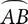is 2π, what is the radian measure of the central angle between A and B ?

• A.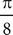• B.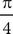• C.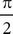• D.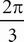C Sketch a picture and write the formulas for arc length and area: s = rθ, A = πr2. Start by finding the radius. If the circle has an area of 16π, then 16π = πr2; divide both sides by π to get 16 = r2. The radius must be 4. So plug in the arc length and radius into your formula: 2π = 4θ. Divide both sides by 4 to get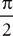= θ. Choice (C) is correct.